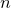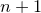# 11.20 fft

```fft { <range> } <function name>()
of ( <filename> | <function name>() )
[ using { <expression> } ]

ifft { <range> } <function name>()
of ( <filename> | <function name>() )
[ using { <expression> } ]
```

The fft command calculates Fourier transforms of data files or functions. Transforms can be performed on datasets with arbitrary numbers of dimensions. To transform an algebraic expression withdegrees of freedom, it must be wrapped in a function of the form. To transform an-dimensional dataset stored in a data file, the samples must be arranged on a regular linearly-spaced grid and stored in row-major order. For each dimension of the transform, a range specification must be provided to the fft command in the form

```[ <minimum> : <maximum> : <step> ]
```

When data from a data file is being transformed, the specified range(s) must precisely match those of the samples read from the file; the firstcolumns of data should contain the values of thereal-space coordinates, and theth column should contain the data to be transformed. After the range(s), a function name should be provided for the output transform: a function ofarguments with this name will be generated to represent the transformed data. Note that this function is in general complex – i.e. it has a non-zero imaginary component. Complex numerics can be enabled using the set numerics complex command and the fft command is of little use without doing so. The using, index, every and select modifiers can be used to specify how data will be sampled from the input function or data file in an analogous manner to how they are used in the plot command.

The ifft command calculates inverse Fourier transforms; it has the same syntax as the fft command.